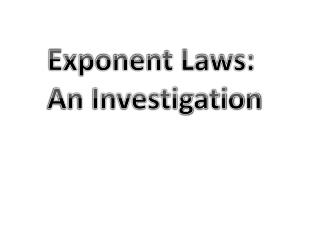DownloadDownload PresentationExponent Laws: An Investigation

# Exponent Laws: An Investigation

Télécharger la présentation## Exponent Laws: An Investigation

- - - - - - - - - - - - - - - - - - - - - - - - - - - E N D - - - - - - - - - - - - - - - - - - - - - - - - - - -
##### Presentation Transcript

1. Exponent Laws: An Investigation

2. 1st Law: Product of Powers Write the expanded form of the first product in the table.

3. 1st Law: Product of Powers Write the expanded form of the first product in the table.

4. 1st Law: Product of Powers Now write it as a single power.

5. 1st Law: Product of Powers Now write it as a single power.

6. 1st Law: Product of Powers Repeat the previous two instructions for the remaining products in the table.

7. 1st Law: Product of Powers Repeat the previous two instructions for the remaining products in the table.

8. 1st Law: Product of Powers Repeat the previous two instructions for the remaining products in the table.

9. 1st Law: Product of Powers Repeat the previous two instructions for the remaining products in the table.

10. 1st Law: Product of Powers Repeat the previous two instructions for the remaining products in the table.

11. 1st Law: Product of Powers Repeat the previous two instructions for the remaining products in the table.

12. 1st Law: Product of Powers Repeat the previous two instructions for the remaining products in the table.

13. 1st Law: Product of Powers Repeat the previous two instructions for the remaining products in the table.

14. 1st Law: Product of Powers Repeat the previous two instructions for the remaining products in the table.

15. What pattern can be seen?

16. In General: When multiplying powers with the same base, keep the base and add the exponents.

17. 2nd Law: Quotient of Powers For each quotient, write both its parts in expanded form and then as a single power.

18. 2nd Law: Quotient of Powers For each quotient, write both its parts in expanded form and then as a single power.

19. 2nd Law: Quotient of Powers For each quotient, write both its parts in expanded form and then as a single power.

20. 2nd Law: Quotient of Powers For each quotient, write both its parts in expanded form and then as a single power.

21. 2nd Law: Quotient of Powers For each quotient, write both its parts in expanded form and then as a single power.

22. 2nd Law: Quotient of Powers For each quotient, write both its parts in expanded form and then as a single power.

23. 2nd Law: Quotient of Powers For each quotient, write both its parts in expanded form and then as a single power.

24. 2nd Law: Quotient of Powers For each quotient, write both its parts in expanded form and then as a single power.

25. 2nd Law: Quotient of Powers For each quotient, write both its parts in expanded form and then as a single power.

26. 2nd Law: Quotient of Powers For each quotient, write both its parts in expanded form and then as a single power.

27. 2nd Law: Quotient of Powers For each quotient, write both its parts in expanded form and then as a single power.

28. What pattern can be seen?

29. In General: When dividing powers with the same base, keep the base and subtract the exponents.

30. 3rd Law: Powers of Powers Write each power of a power in expanded form and then as a single power.

31. 3rd Law: Powers of Powers Write each power of a power in expanded form and then as a single power.

32. 3rd Law: Powers of Powers Write each power of a power in expanded form and then as a single power.

33. 3rd Law: Powers of Powers Write each power of a power in expanded form and then as a single power.

34. 3rd Law: Powers of Powers Write each power of a power in expanded form and then as a single power.

35. 3rd Law: Powers of Powers Write each power of a power in expanded form and then as a single power.

36. 3rd Law: Powers of Powers Write each power of a power in expanded form and then as a single power.

37. What pattern can be seen?

38. In General: When writing a power of a power as a single power, keep the base and multiply the exponents.

39. Try a few examples…

40. Write each as a single power, then evaluate using a calculator. a) b) c)

41. Write each as a single power, then evaluate using a calculator. a) b) c)

42. Write each as a single power, then evaluate using a calculator. a) b) c)

43. Write each as a single power, then evaluate using a calculator. a) b) c)

44. Write each as a single power, then evaluate using a calculator. a) b) c)

45. Write each as a single power, then evaluate using a calculator. a) b) c)

46. Write each as a single power, then evaluate using a calculator. a) b) c)

47. Now try these…

48. Simplify. a) b) c)

49. Simplify. a) b) c)

50. Simplify. a) b) c)